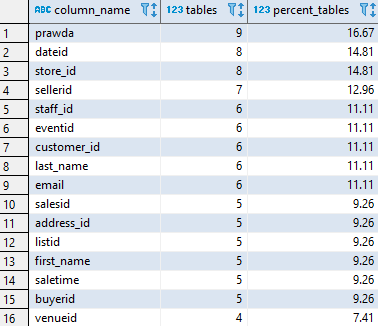## Find most popular column names in Redshift

Article for:

This query lets you find out most popular column names.

## Query

``````select c.column_name,
count(*) as tables,
round(100.0*count(*)::decimal
/(select count(*)as tables
from information_schema.tables
where table_type = 'BASE TABLE'
and table_schema not in ('information_schema', 'pg_catalog'))
, 2) as percent_tables
from information_schema.columns c
join information_schema.tables t
on t.table_schema = c.table_schema
and t.table_name = c.table_name
where t.table_type = 'BASE TABLE'
and t.table_schema not in ('information_schema', 'pg_catalog')
group by c.column_name
having count(*) > 1
order by count(*) desc;
``````

## Columns

• column_name - column name
• tables - number of tables that have particular column name
• percent_tables - percentage of tables with column with that name

## Rows

• One row represents particular column name (each name appears only once)
• Scope of rows: all colum names that appear at least twice (in two tables)
• Ordered by the most popular first

## Sample results